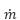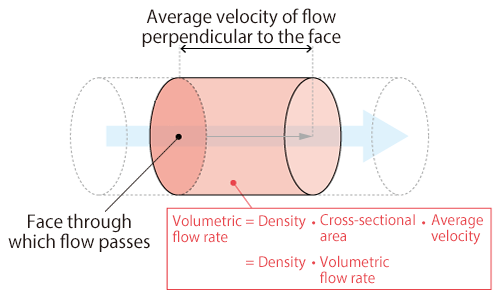#1. TOP
2. Resource Library
3. CAE Glossary
4. K-O index
5. Mass flow rate

# Mass flow rate

## Mass flow rate

Mass flow rate is the mass of fluid that passes through a certain face per unit time.

When fluid density is constant, mass flow rate can be expressed with the following equation:where;: Mass flow rate [kg/s]
ρ  : Fluid density [kg/m3]
A  : Area of the face through which flow passes through[m2]
v  : Average fluid velocity of flow perpendicular to the face through which fluid passes [m/s]

The equation above can also be expressed using volumetric flow rate Q [m3/s] as below: